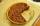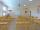# Camp

In the camp are children. 1/2 went on a trip, 1/4 went to bathe and 38 children remained in the room. How many children are in camp?

Result

x =  152

#### Solution:Leave us a comment of example and its solution (i.e. if it is still somewhat unclear...):Be the first to comment!#### To solve this example are needed these knowledge from mathematics:

Need help calculate sum, simplify or multiply fractions? Try our fraction calculator. Do you have a linear equation or system of equations and looking for its solution? Or do you have quadratic equation?

## Next similar examples:

1. ClassroomOne-eighth of 9th class was interested in studying at a grammar school, at a business academy one sixth, at secondary vocational schools quarter, to SOU one third and the remaining three students were interested in the school of art direction. How many st
2. Fractions 4How many 2/3s are in 6?
3. Trees3/5 trees are apples, cherries are 1/3. 5 trees are pear. How many is the total number of trees?
4. Lengths of the poolMiguel swam 6 lengths of the pool. Mat swam 3 times as far as Miguel. Lionel swam 1/3 as far as Miguel. How many lengths did mat swim?
5. Passenger boatTwo-fifths of the passengers in the passenger boat were boys. 1/3 of them were girls and the rest were adult. If there were 60 passengers in the boat, how many more boys than adult were there?
6. Pizza 4Marcus ate half pizza on monday night. He than ate one third of the remaining pizza on Tuesday. Which of the following expressions show how much pizza marcus ate in total?
7. Pie IIVili ate three pieces of pie. If it pieces is 1/8 how much pie did he eat?
8. Watching TVOne evening 2/3 students watch TV. Of those students, 3/8 watched a reality show. Of the students that watched the show, 1/4 of them recorded it. What fraction of the students watched and recorded reality tv.
9. Classroom 4In a class of 36 pupils, 2/3 are girls. How much it is in a class girls and boys?
10. In fractionsAn ant climbs 2/5 of the pole on the first hour and climbs 1/4 of the pole on the next hour. What part of the pole does the ant climb in two hours?
11. Number with onesThe first digit of the number is 1, if we move this digit to the end we get a 3 times higher number, which is the number?
12. Find xSolve: if 2(x-1)=14, then x= (solve an equation with one unknown)
13. Dropped sheetsThree consecutive sheets dropped from the book. The sum of the numbers on the pages of the dropped sheets is 273. What number has the last page of the dropped sheets?
14. ZdeněkZdeněk picked up 15 l of water from a 100-liter full-water barrel. Write a fraction of what part of Zdeněk's water he picked.
15. Simple equation 5Solve equation with fractions: X × 3/8 = 1/2
16. Equation 11Solve equation: ?
17. Find unknownFind unknown numerator: 4/8 + _/8 = 1